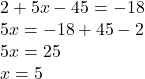## Solve the equation and enter the value of x below. 2 + 5(x – 9) = -18​

Question

Solve the equation and enter the value of x below. 2 + 5(x – 9) = -18​

in progress 0
7 months 2021-07-27T03:33:16+00:00 2 Answers 5 views 0